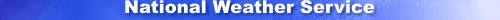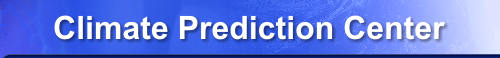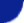Site Map News Organization SearchHOME > Monitoring_and_Data > Oceanic and Atmospheric Data > Reanalysis: Atmospheric Data > wgrib2-pds_fcst_time

Introduction

Most grib messages include a forecast time which is stored in the Product Definition Templates (PDT). When the PDT has a forecast time, it stores the number as a 4 byte integer and the units in Code Table 4.4. For example, a 12 hour forecast could be stored with the number 12 and the units of 1 which corresponds hour. Note that the same forecast time can be stored in several ways. For example, 12 hours can be stored as

• value=720 , units = 0 (minutes)
• value=12 , units = 1 (hours)
• value=4 , units = 10 (3-hours)
• value=2 , units = 11 (6-hours)
• value=1 , units = 12 (12-hours)
• value=43299, units = 13 (seconds)
The unusual 3-hour, 6-hour and 12-hour time units are a legacy of the grib version 1 standard. Note that the value is a signed integer because the grib standard allows negative forecast hours which can make sense in data assimilation.

The -pds_fcst_time option prints out the value of the forecast time and the -code_table_4.4 option prints out the units of the forecast time.

-pds_fcst_time

Example

\$ wgrib2 percentile_precip.grib2 -s -pds_fcst_time -code_table_4.4
1:0:d=2014101012:TPRATE:surface:2@1 hour max(13-14 hour acc fcst)++,missing=0:75% level:pds_fcst_time1=13:code table 4.4=1 (hour)
2:315649:d=2014101012:TPRATE:surface:2@1 hour max(13-14 hour acc fcst)++,missing=0:90% level:pds_fcst_time1=13:code table 4.4=1 (hour)